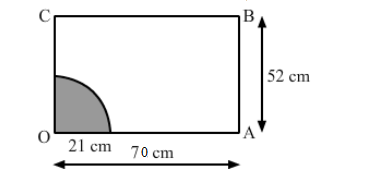# A horse is placed for grazing inside a rectangular field 70 m by 52 m.`
Question:

A horse is placed for grazing inside a rectangular field 70 m by 52 m. It is tethered to one corner by a rope 21 m long. On how much area can it graze? How much area is left ungrazed?

Solution:

The shaded portion shows the part of the field the horse can graze.Area of the grazed field = Area of the quadrant OPQ

$=\frac{1}{4}$ of the circle having radius OP

$=\frac{1}{4} \pi \mathrm{r}^{2}$

$=\frac{1}{4} \times \frac{22}{7} \times 21 \times 21$

$=346.5 \mathrm{~m}^{2}$

Total area of the field $=70 \times 52=3640 \mathrm{~m}^{2}$

Area left ungrazed = Area of the field - Area of the grazed field

$=3640-346.5=3293.5 \mathrm{~m}^{2}$